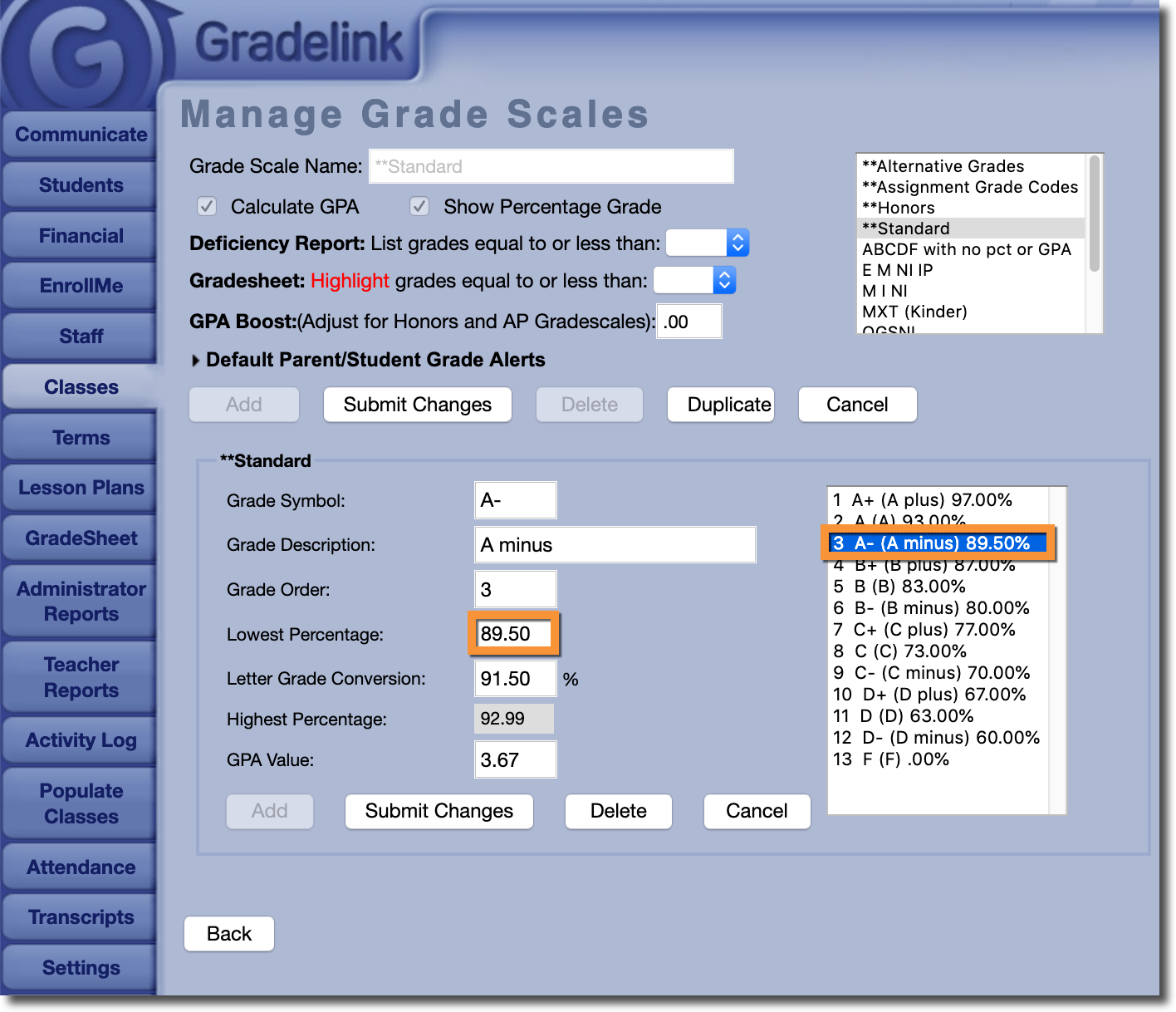Possible Scenario:

How does Gradelink round grades?

Why aren't my grades rounding?

Solution:

Gradelink calculates grades based on the grade scale applied to each class. Grade scales in Gradelink require upper and lower numeric bounds in order to convert letter grades to percentages and vice versa.

Therefore, Gradelink does not automatically round up grades that are on the cusp of another higher or lower grade. The only exception is "Double Rounding" on Report Cards; you can read more about "Double Rounding" here.

If you would like to enable rounding on assignments and overall grades (as in, you would like grades that are within a certain margin from a higher or lower grade to be 'bumped' to that grade), you can do so by modifying the Highest and Lowest Percentages on the grade scale assigned to a class.

For example, let's assume that on a particular grade scale, an "A-" has a Lowest Percentage of 90.0%, and a "B+" has a Highest Percentage of 89.99%. If you would like a grade such as an 89.5% that would normally qualify as a "B+" to 'round up' to an "A-", you would need to adjust the Lowest Percentage for an "A-" to be 89.5% (the Highest Percentage for each grade is calculated automatically, so you don't have to worry about adjusting it).

With this change in place, grades such as an 89.5% would now 'round up' to an "A-".